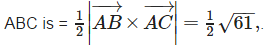Courses

# Test: Vector Algebra (CBSE Level) - 2

## 25 Questions MCQ Test Mathematics (Maths) Class 12 | Test: Vector Algebra (CBSE Level) - 2

Description
This mock test of Test: Vector Algebra (CBSE Level) - 2 for JEE helps you for every JEE entrance exam. This contains 25 Multiple Choice Questions for JEE Test: Vector Algebra (CBSE Level) - 2 (mcq) to study with solutions a complete question bank. The solved questions answers in this Test: Vector Algebra (CBSE Level) - 2 quiz give you a good mix of easy questions and tough questions. JEE students definitely take this Test: Vector Algebra (CBSE Level) - 2 exercise for a better result in the exam. You can find other Test: Vector Algebra (CBSE Level) - 2 extra questions, long questions & short questions for JEE on EduRev as well by searching above.
QUESTION: 1

### Zero Vector

Solution:

A vector whose initial and terminal points coincides is called Zero Vector. The magnitude of Zero Vector is also Zero.

QUESTION: 2

### If a vector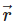is expressed in component form as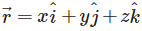then the magnitude of the vectoris

Solution: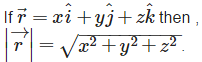QUESTION: 3

### Find the value of x for which x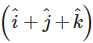is a unit vector

Solution: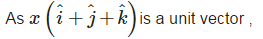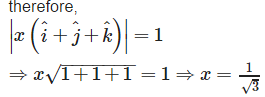QUESTION: 4

Find the angle between two vectors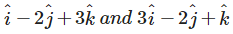Solution: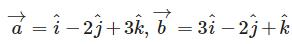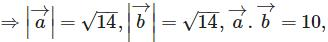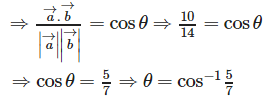QUESTION: 5

Find λ and μ if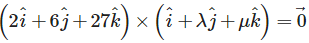Solution:

It is given that: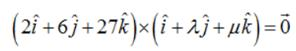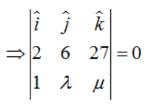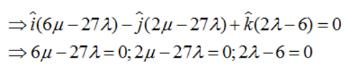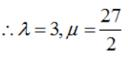QUESTION: 6

Unit Vector is

Solution:

The vector whose magnitude is always 1 or unity is called a Unit Vector.

QUESTION: 7

If a vector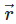is expressed in component form as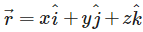then x, y and z are referred to as

Solution:

Inx , y and z represents the components ofalong x-axis ,y-axis and z-axis respectively.

QUESTION: 8

Show that the points A(1, – 2, – 8), B (5, 0, – 2) and C (11, 3, 7) are collinear, and find the ratio in which B divides AC.

Solution: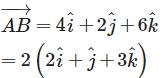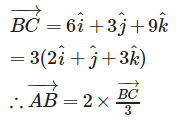Therefore , AB and BC are parallel, but point B is common , so points , A,B,C are collinear. As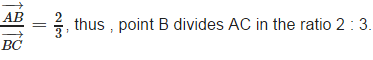QUESTION: 9

Find the projection of the vector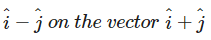Solution: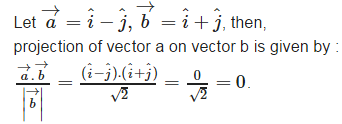QUESTION: 10

Let the vectors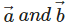be such that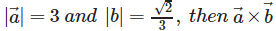is a unit vector if the angle between vectorsis

Solution: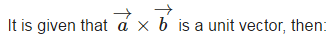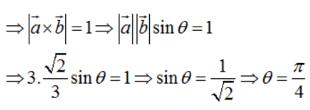QUESTION: 11

The unit vector in the direction of a given vector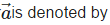Solution: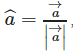is called the unit vector of a given vector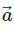QUESTION: 12

Which of the following is a vector?

Solution:

Acceleration is a vector quantity , because acceleration is defined as the rate of change of velocity w.r.t. time.

QUESTION: 13

Find the position vector of a point R which divides the line joining two points P and Q whose position vectors are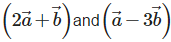externally in the ratio 1 : 2. Also, show that P is the mid point of the line segment RQ.

Solution:

Let position vector of point R be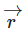. As point R divides externally the line segment PQ in the ratio 1:2 .therefore ,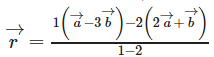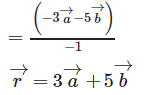Also , mid point of the line segment RQ is :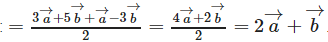Which is the position vector of point P.
Therefore , P is the mid point of line segment RQ.

QUESTION: 14

Find the projection of the vector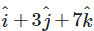on the vector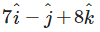Solution: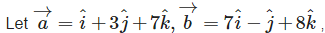then projection of vector a on vector b is given by :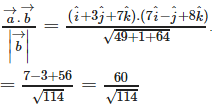QUESTION: 15

If θ is the angle between two vectors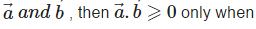Solution: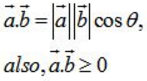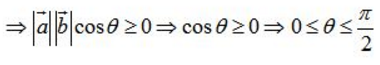QUESTION: 16

Coinitial Vectors are

Solution:

Two vectors whose initial point is same are called co- initial vectors

QUESTION: 17

In the figure which are the Equal vectors?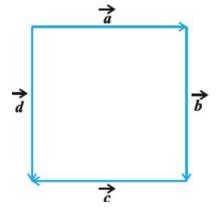Solution: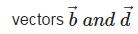are equal vectors , because they have equal magnitude and same direction.

QUESTION: 18

The scalar product of two nonzero vectors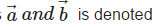Solution:

The scalar product of two nonzero vectorsby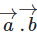QUESTION: 19

Find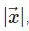if for a unit vector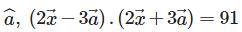Solution: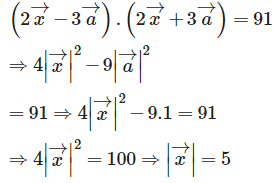QUESTION: 20

If θ is the angle between any two vectors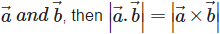when θ is equal to

Solution: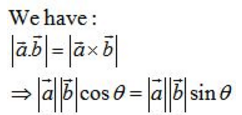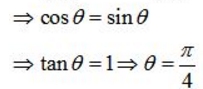QUESTION: 21

Vectors A and BareCollinear

Solution:

Two vectors A and B are said to be collinear , if they are parallel to the same line irrespective of their magnitudes and directions.

QUESTION: 22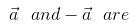Solution: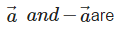collinear vectors , because they are parallel in direction and having the same magnitude.

QUESTION: 23

The scalar product of two nonzero vectors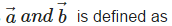Solution:

The scalar product of two nonzero vectors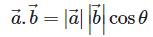QUESTION: 24

If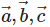are unit vectors such that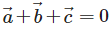then, the value of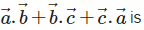Solution:

It is given that :are unit vectors such thatthen :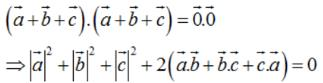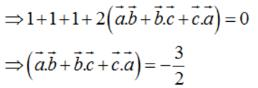QUESTION: 25

Find the area of the triangle with vertices A(1, 1, 2), B(2, 3, 5) and C(1, 5, 5).

Solution: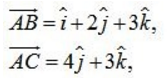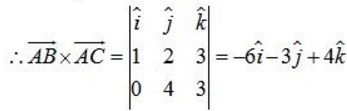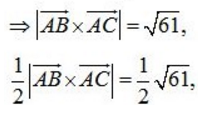Therefore , the area of triangle